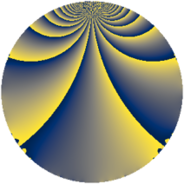# Properties

 Label 546.2.bqLevel $546$ Weight $2$ Character orbit 546.bq Rep. character $\chi_{546}(419,\cdot)$ Character field $\Q(\zeta_{6})$ Dimension $72$ Newform subspaces $3$ Sturm bound $224$ Trace bound $6$

# Related objects

## Defining parameters

 Level: $$N$$ $$=$$ $$546 = 2 \cdot 3 \cdot 7 \cdot 13$$ Weight: $$k$$ $$=$$ $$2$$ Character orbit: $$[\chi]$$ $$=$$ 546.bq (of order $$6$$ and degree $$2$$) Character conductor: $$\operatorname{cond}(\chi)$$ $$=$$ $$273$$ Character field: $$\Q(\zeta_{6})$$ Newform subspaces: $$3$$ Sturm bound: $$224$$ Trace bound: $$6$$ Distinguishing $$T_p$$: $$5$$, $$61$$

## Dimensions

The following table gives the dimensions of various subspaces of $$M_{2}(546, [\chi])$$.

Total New Old
Modular forms 240 72 168
Cusp forms 208 72 136
Eisenstein series 32 0 32

## Trace form

 $$72q + 36q^{4} + 8q^{7} + 4q^{9} + O(q^{10})$$ $$72q + 36q^{4} + 8q^{7} + 4q^{9} + 12q^{15} - 36q^{16} - 24q^{18} + 16q^{21} + 56q^{25} - 8q^{28} + 8q^{30} - 4q^{36} - 16q^{37} - 48q^{39} + 8q^{42} - 12q^{43} - 4q^{46} + 4q^{49} + 16q^{51} + 8q^{57} - 8q^{58} + 24q^{60} + 8q^{63} - 72q^{64} + 52q^{67} - 12q^{72} + 36q^{78} + 16q^{79} + 32q^{81} + 8q^{84} - 88q^{85} - 64q^{91} - 56q^{93} + O(q^{100})$$

## Decomposition of $$S_{2}^{\mathrm{new}}(546, [\chi])$$ into newform subspaces

Label Dim. $$A$$ Field CM Traces $q$-expansion
$$a_2$$ $$a_3$$ $$a_5$$ $$a_7$$
546.2.bq.a $$4$$ $$4.360$$ $$\Q(\zeta_{12})$$ None $$0$$ $$0$$ $$0$$ $$2$$ $$q+\zeta_{12}q^{2}+(-\zeta_{12}+2\zeta_{12}^{3})q^{3}+\zeta_{12}^{2}q^{4}+\cdots$$
546.2.bq.b $$4$$ $$4.360$$ $$\Q(\zeta_{12})$$ None $$0$$ $$0$$ $$0$$ $$-10$$ $$q+\zeta_{12}q^{2}+(\zeta_{12}-2\zeta_{12}^{3})q^{3}+\zeta_{12}^{2}q^{4}+\cdots$$
546.2.bq.c $$64$$ $$4.360$$ None $$0$$ $$0$$ $$0$$ $$16$$

## Decomposition of $$S_{2}^{\mathrm{old}}(546, [\chi])$$ into lower level spaces

$$S_{2}^{\mathrm{old}}(546, [\chi]) \cong$$ $$S_{2}^{\mathrm{new}}(273, [\chi])$$$$^{\oplus 2}$$